# Acid evaporation

How many kilograms of water do we have to evaporate from 100 kg of 32% acid to make it 80% concentration?

m =  60 kg

### Step-by-step explanation:

0.80•(100-m) = 0.32•100

0.8m = 48

m = 60

Our simple equation calculator calculates it.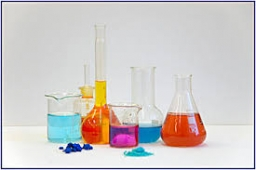Did you find an error or inaccuracy? Feel free to write us. Thank you!Tips to related online calculators
Do you have a linear equation or system of equations and looking for its solution? Or do you have a quadratic equation?

## Related math problems and questions:

• Alcohol mixtureFrom 55% and 80% alcohol, we have to produce 0.2 kg of 60% alcohol. How many of them do we use in the solution?
• Apples 2Dried apples contain 15% water. Fresh apples contain 80% water. How many kg of apples we need buy in order to get 3 kg of dried apples?
• MushroomsFresh mushrooms contain 88% water, dried 14% water. How many kilograms of fresh mushrooms should be collected to get 3 kg of dried?
• SpiritFrom 55% and 80% spirit we would like to produce 0.2 kg of 60% spirit. How many of them we must use in a solution?
• Sea waterMixing 62 kg of sea water with 84 kg rainwater is created water containing 3.1% salt. How many percent sea water contains salt?
• Acid solutionBy adding 250 grams of a 96% sulfuric acid solution to its 3% solution, we changed its initial concentration to 25%. How many grams of 3% of the acid was used for dilution?
• Distilled waterSea water contains 5% salt. How many distilled water should be pour to 40 kg of sea water so that the salt content is 2%. How many kilograms of 2% of sea water we get?
• Coffee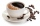One kilogram of cheaper coffee costs CZK 150, one kilogram of more expensive coffee costs CZK 200. We have to prepare a mixture of 35 kg of coffee for 180 CZK. How do we prepare the mixture (how many of which kind of coffee do we need)?
• ConcentrationHow many grams of 65% and 50% acid is to be mixed to give 240 g acid with a concentration of 60%?
• Three piles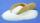100 kg of sugar we divide into three piles. The first pile is small. If we added to second 2 kg of sugar it would have 25% more sugar than the first pile. If we added to third pile 3 kg of sugar it would have 20% more sugar than the 2nd pile. How many kil
• GramsHow many grams of 5% solution do we need to add to 100g of 50% solution to get a 20% solution?
• Acids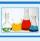70% acid made from the same acid of two different concentrations. Amount weaker acid to the stronger acid is in ratio 2:1. What was the concentration of the weaker acid if stronger had 91% concentration?
• Solutions, mixturesWe have 2 liters of 20% solution available. How much 70% solution do we need to add to it to get a 30% solution?
• Camel and water84% of the camel's weight is water. After drinking, its weight increased to 832 kg, and water accounted for 85% of its weight. How much did it weigh before drinking?
• Copper sulphate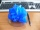How much g of water do we have to add to 240 g of an 84% CuSO4 solution to produce a 60% solution? (Express the mass of crystalline CuSO4 in the original solution and in the resulting solution and compare them. )
• SaltSeawater containing 5% salt. How many kg of freshwater necessary to pour into 40 kg of seawater so that the salinity fell to 2%?
• Alcohol solutionsWe have to produce 2 liters of 60% alcohol from 55% and 80%. How many of which ones will we use in the solution?